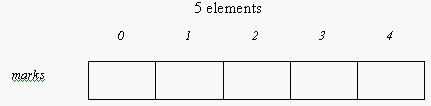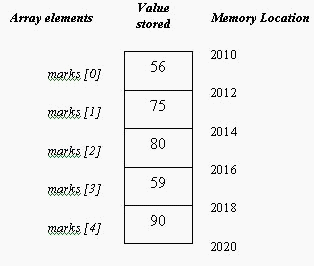More On Data Types and Variables

The following topics are covered in this section:

Type Qualifiers

Variables can be given a qualifier (something that precedes their data type) to specify how they will behave in the program. The two qualifiers that can be used are:

• const (constant)
• volatile

They are sometimes referred to as type-qualifiers (since they specify how the program can modify these variables).

Constants

Constants are fixed and will never change (i.e. their value will never change). There might be occasions when the programmer will want to make use of variables whose value should never be modified within the program. When we use variables (As we have done so far in this book), we can change the value of the variable whenever we want to. But once a variable is declared to be a constant (using the keyword ‘const’), it’s value cannot be altered in the program. For example:

const float PI=3.14;

will make PI a constant with the value of 3.14. A constant should always be initialized with a value (you cannot simply declare const float PI; A value has to be assigned to it as well).

By the way, what do you call ‘pi’ in the above example? Is it called a constant variable? Some people use the term ‘constant variable’ (perhaps as a way of distinguishing them from the constants that we saw in the second chapter). Actually this term is an oxymoron. It is advisable to use the term variable when you refer to something whose value can be changed while the term constant is used whenever the value cannot be altered. So ‘PI’ is a constant.

Remember: Programmers usually use uppercase identifiers (example: PI, MAX etc.) for constants while lowercase identifiers (like x, marks, age etc.) are used for variables.

There is another way to declare constants (this method was used in C programming). Instead of the ‘const’ keyword, we can make use of macros.

#include <iostream.h>
#define PI 3.14                     //Macro definition
int main( )
{
cout<<PI;
return 0;
}

The output would be:

3.14

# indicates that the statement is a preprocessor directive. This topic will be explained in detail later in the book. In the above program PI is called a ‘macro’. Wherever the term PI is encountered, the number 3.14 will be substituted in its place.

Remember: C++ is a case-sensitive language, which means that the compiler will not consider PI and ‘pi’ as one and the same.

Beware: You shouldn’t use the equal to sign in the preprocessor directive statement and neither should you use the semicolon terminator at the end.

Volatile

The volatile keyword is the opposite of ‘const’. The volatile keyword informs the compiler that the variable can be modified by methods that are unseen (or external). For instance, this external method could be an interrupt-related method. If we don’t specify the variable as volatile, then the compiler might end up denying modification of the variable by such an external method. To declare a variable as volatile, just precede the declaration by the keyword ‘volatile’.

The syntax is:

volatile data-type variable-name;

Variable Initialization

Assigning values to a variable at the time of declaration is known as variable initialization.

For example:

int x = 10;

double db=2e4;

If global or static variables are not initialized they are automatically set to zero. If a local variable is declared and not assigned any value then it will contain an unknown value (i.e. you can’t say for sure what is the value of the variable. This is sometimes termed as ‘garbage’ value).

For example:

int main( )
{
int local;
cout<<local;                     //value is unpredictable. It can have any value.
return 0;
}

Remember: Local variables will contain an unknown value till they are assigned a value.

There is another way to initialize variables (instead of using the = operator). The following initialization is also valid in C++:

int count(5);

This will initialize the variable ‘count’ to 5. It is the same as:

int count=5;

Arrays

If you want to use a variable you should declare the variable first. What if you need to obtain the marks of a student in 20 subjects? Should you make use of 20 variables? You could write the code as:

int marks1, marks2, marks3…;

This would make the process of declaring variables and obtaining inputs very tedious. Arrays provide an easier way of working with many variables, which are logically related. An array consists of a set of variables of the same data type. Each variable of an array is called its element and each of the elements can be accessed using subscript numbers (or index numbers).

The difference between an array and a structure is that array consists of a set of variables of the same data type. A structure, on the other hand, can consist of different data types. You'll learn about structures later on.

The first step if you want to use an array is declaration of the array.

data-type variable-name [size-of-array];

For example:

int marks;

This means that you have declared an array of integer type. The variable name for the array is ‘marks’ and size of the array is 5. Hence you have actually declared five integer elements namely: marks , marks , marks , marks and marks.

You may have a question at this point: What about marks?

The computer counts from zero onwards. The size of the array we declared is 5. So if you start counting from zero then the fifth element is marks not marks.If an integer occupies 2 bytes, then the ‘marks’ array declared above will occupy a total of 10 bytes continuously. Each individual element can store an integer value. The figure below illustrates this concept:Remember: Start counting from zero. Many beginners do not pay attention to this important fact.

1. Array is a collection of variables (of the same data type).
2. Each individual element is denoted by its index number.
3. Array elements are placed consecutively in memory (in other words array elements occupy contiguous memory locations; i.e. they are placed adjacent to one another).
4. The size of the array has to be specified while declaring the array (because the compiler has to allocate memory for the individual elements).

Accessing a particular element:

If you want to display the values of an array, you might think that this will work:

cout<<marks; //WRONG

This is wrong! It seems as if this is fine but this will not work. There is no way that you can display the entire contents of an array by simply specifying its common name. You can print the individual elements as below:

cout<<marks;

cout<<marks;

Similarly if you want to assign a value to one of the elements, you would write the following code:

marks = 56;

marks = 90;

Just type the variable name followed by the element number within the square brackets. Be careful with the type of bracket; it’s a square bracket and not the curly braces.

Then you might be wondering what’s the big deal of using arrays? Since to access individual elements only the index number has to be changed, we can easily display the values stored in an array by using a ‘for’ loop. Another advantage is that the name of the array is the same. Otherwise you would have to use different identifiers.

Initializing an Array:

Suppose you want to initialize the array element values at the beginning itself (during the declaration) then you could make use of a comma-separated list within braces as shown below:

int marks = {56, 75, 80, 59, 90};

This statement tells the compiler that the value of the first element of the array (or marks ) is 56, the second element ( marks ) is 75 and so on. The initialization of an array is equivalent to:

marks=56;
marks=75;
marks=80;
marks=59;
marks=90;

While initializing an array the number of elements initialized must be equal to the size of the array. In the above example we have 5 elements and all 5 elements have been initialized in the statement:

int marks = {56, 75, 80, 59, 90};

Instead of this we could also initialize using the statement:

int marks[ ] = {56, 75, 80, 59, 90};

The compiler will automatically set the size of the array as 5 (because 5 elements have been initialized).

Remember: For the initialization you have to use curly braces and for the index numbers you have to use square brackets.

Memory usage for an array:

If you have an array of 10 short integers, then the array will occupy a total of 2*10 bytes (20 bytes). An array of 10 long integers would occupy 4*10 bytes (40 bytes).

double temp;

cout<<sizeof(temp);

will produce an output of 40 (because each double variable will occupy 8 bytes). But what if we tried:

double temp;

cout<<sizeof(temp);

This would return the size of the 5th element; which means that the output will be 8.

Apply what you’ve learnt

Let’s write a program that utilizes the concept of arrays. We’ll write a program to arrange a set of given numbers in ascending order and display the result. The basic steps involved will be:

Obtain the size of the array (i.e. how many numbers the user wants tosort, 2 numbers or 3 or 4 or 8 numbers etc.)

Obtain the value of each element of the array. (Hint: make use of a for loop)

Compare the values of each element with the others and sort the numbers.

//To arrange a given set of numbers in ascending order and display the result

#include <iostream.h>
int main ( )
{int test; //we assume that the maximum limit is 20 numbers.
int size,i,j;
int temp;
cout<<"How many numbers do you want to compare : ";
cin>>size;
cout<<"Enter the numbers you want to check : ";
for(i = 0; i<size; i ++) //obtain the numbers
{
cin>>test[ i ];
}
for(i = 0; i<size; i++) //2 loops for sorting the numbers
{
for (j = 0; j<size; j++)
{
if ( test[i]>test[j] )
{
temp = test[ i ];
test[ i ] = test[ j ];
test[ j ] = temp;
}
}
}
cout<<"The numbers in ascending order are : ";
for (i = 0; i<size; i ++) // loop used for displaying sorted values
{
cout<<endl<<test[ i ];
}
return 0;
}

As can be seen from the program, it is much easier to obtain inputs for array elements as well as displaying the values using loops. By the way, the above method of sorting is simple to implement but quite slow. There are other methods like the quicksort method, which will consume less time.

Go back to the Contents Page